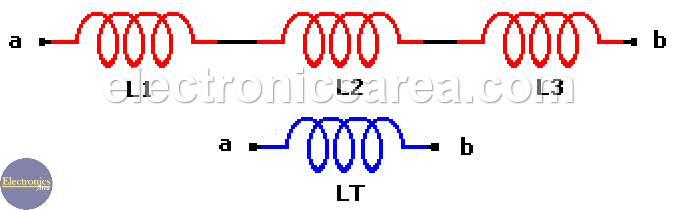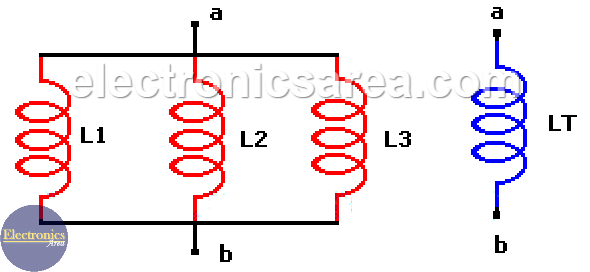# Series and Parallel Inductors – Series & Parallel equivalent inductance

On many occasions it is necessary to obtain the equivalent value of a set of inductors that are connected in series or parallel.

This are the simplest methods to calculate the values (inductance) of series and parallel inductors.

## Inductors in series

The calculation of the equivalent inductor of a set of inductors in series is similar to the calculation of the equivalent resistor of a set of resistors that are in series, then you just need to add their values.

The diagram below shows 3 inductors in series and the formula used here is: LT = L1 + L2 + L3.

This formula works fine for the 3 inductors in series of the diagram, but if we have any number of inductors is better to use the following formula: LT = L1 + L2 + L3 +……+ LN, where N is the number  of inductors in series.Example 1:

If we have 3 inductors with the following values:

• L1 = 10 mH
• L2 = 20 mH
• L3 = 30 mH

The equivalent inductor is: LT = L1 + L2 + L3 = 10 mH + 20 mH + 30 mH = 60 mH

Example 2:

If we have 4 inductors with the following values:

• L1 = 5 mH
• L2 = 40 mH
• L3 = 20 mH
• L4 = 30 mH

The equivalent inductor is: LT = L1 + L2 + L3 + L4 = 5 mH + 40 mH + 20 mH + + 20 mH = 95 mH

## Inductors in parallel

The calculation of the equivalent inductor of a set of inductors in parallel is similar to the calculation of the equivalent resistor of a set of resistors that are in parallel.The diagram above shows 3 inductors in parallel and the formula used is:  1/LT = 1/L1 + 1/L2 + 1/L3.

This formula works fine for the 3 inductors in parallel of the last diagram, but if we have any number of inductors, we can use the formula: 1/LT = 1/L1 + 1/L2 + 1/L3 + …. 1/LN, where N is the number of inductors connected in parallel.

Example 3:

• L1 = 20 mH
• L2 = 100 mH
• L3 = 50 mH

The equivalent inductor in parallel is:

1/LT = 1/L1 + 1/L2 + 1/L3 = 1/20 mH + 1/100 mH + 1/50 mH = 0.05 + 0.01 + 0.02 = 0.08.

1/LT = 0.08, then LT = 1/0.08 = 12.5 mH

Example 4:

• L1 = 100 mH
• L2 = 100 mH
• L3 = 100 mH
• L4 = 100 mH

The equivalent inductor in parallel is:

1 / LT = 1/L1 + 1/L2 + 1/L3 + 1/L4 = 1/100 mH + 1/100 mH + 1/100 mH + 1/100 mH = 0.01 + 0.01 + 0.01 + + 0.01 = 0.04.

1/LT = 0.04, then LT = 1/0.04 = 25 mH

• 3
•
• 1
•
•
•
•
•
•
4
Shares
•
4
Shares
• 3
•
• 1
•
•
•
•
•